# Getting started with Yokogawa data

## Introduction

The datafiles for the 64-, 160- and 440-channel Yokogawa MEG systems are supported by using the precompiled (i.e. closed source) p-files that are supplied by Yokogawa. The data in the following files can be read and used in FieldTrip: .sqd, .ave, .con, .raw. Furthermore, gradiometer positions and orientations are read from the header (see below).

The low-level MATLAB reading functions are included in the FieldTrip release. Note that these files are not open source and not covered by the GPL license, but they are copyrighted by Yokogawa.

FieldTrip includes reading functions for the Yokogawa MEG system in the fieldtrip/external/yokogawa directory. Using those reading functions, FieldTrip can process Yokogawa data just like any other data type. That means that the default reading functions ft_read_header, ft_read_data and ft_read_event work just like expected. The gradiometer information is also correctly represented, which means that you can use FieldTrip or SPM (which uses FieldTrip for the forward computations) for forward and inverse source estimation.

The following Yokogawa file formats are recognized by FieldTrip

• yokogawa_sqd Continuous data
• yokogawa_con Continuous data
• yokogawa_ave Averaged evoked fields
• yokogawa_raw Trial-based evoked fields
• yokogawa_mri
• yokogawa_coregis
• yokogawa_calib
• yokogawa_channel
• yokogawa_property
• yokogawa_textdata
• yokogawa_fll

Usually you will be starting your FieldTrip analysis with raw continuous data which is stored in files with the .con or the .sqd extension.

## Set the path

To get started, you should add the FieldTrip main directory to your path, and execute the ft_defaults function, which sets the defaults and configures up the minimal required path settings. See also this frequently asked question.

``````addpath <path_to_fieldtrip>
ft_defaults
``````

## Testing that the reading into MATLAB works

To test that the reading of the continuous data works on your computer, you can try something like the following on your own data file

``````>> hdr = ft_read_header('Continuous1.con')

hdr =
Fs: 500
nChans: 224
nSamples: 2500
nSamplesPre: 0
nTrials: 1
label: {1x224 cell}
orig: [1x1 struct]
``````

In hdr.label you can find the channel labels (as strings). In hdr.grad you can find the magnetometer and gradiometer definition (i.e. the position of each coil and how the coils are combined into channels).

Subsequently you can read the data from one of the channels (here the first) and simply plot i

``````>> dat = ft_read_data('Continuous1.con', 'chanindx', 1);
>> plot(dat)
``````

Instead of using the low-level reading functions for reading and handling the data manually, normally you would use the ft_definetrial and ft_preprocessing. Please read through the tutorials to learn how to do a complete analysis.

The historical development and continuous push for improvements causes FieldTrip to supports three different codebases to read in Yokogawa file

1. external\yokogawa by the Yokogawa company, old version
2. external\sqdproject by Shantanu Ray, ISR, University of Maryland

The initial implementation was based on the first external toolbox. It turned out that especially on windows computers it was too slow to work efficiently, that is why we looked into an alternative. The second Sqdproject toolbox is more memory and time efficient for reading the data and it can be used in conjunction to the first (for reading the header and meta information) by also adding sqdproject to the MATLAB path.

The third toolbox contains the completely renewed import functions from the Yokogawa company, which they released to us end 2011. This is the one which probably works the best and most (memory and speed) efficient in most cases, that is why this is the default. If you don’t want to use it, please delete it from the fieldtrip/external directory or change its name into “yokogawa_meg_reader_unused” or something similar.

Due to possible differences in e.g., calibration it is strictly recommended to use one codebase consistently for all your analysis.

## Reading triggers into an event-structure

Yokogawa uses analogue channels as trigger channels, often in a binary way. Which is to say that e.g., a 5 volt signal is transiently put on the channel when a stimulus is presented. Often several channels are used to be able to encode several trigger ‘codes’.

This means that the user (you!) needs to know exactly which channels are used, whether a trigger is encoded by an up-flank or down-flank and what the threshold should be. One way of figuring out these is to just take a look at the trigger channel.

``````data = ft_read_data('test.sqd');
``````

We can make a plot of one of the trigger channels

``````figure;
plot(data(161,1:100000);
``````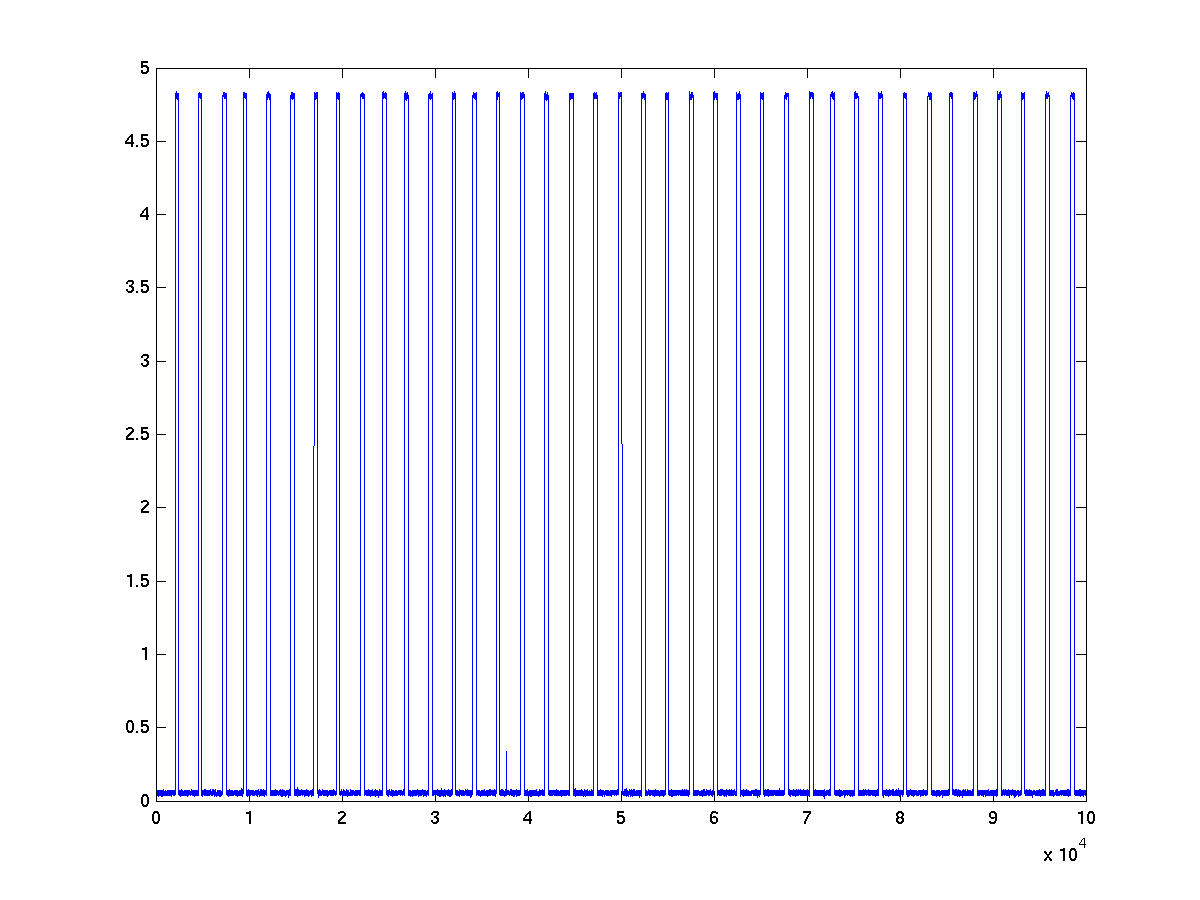zooming in a bit

``````figure;
plot(data(161,1:10000);
``````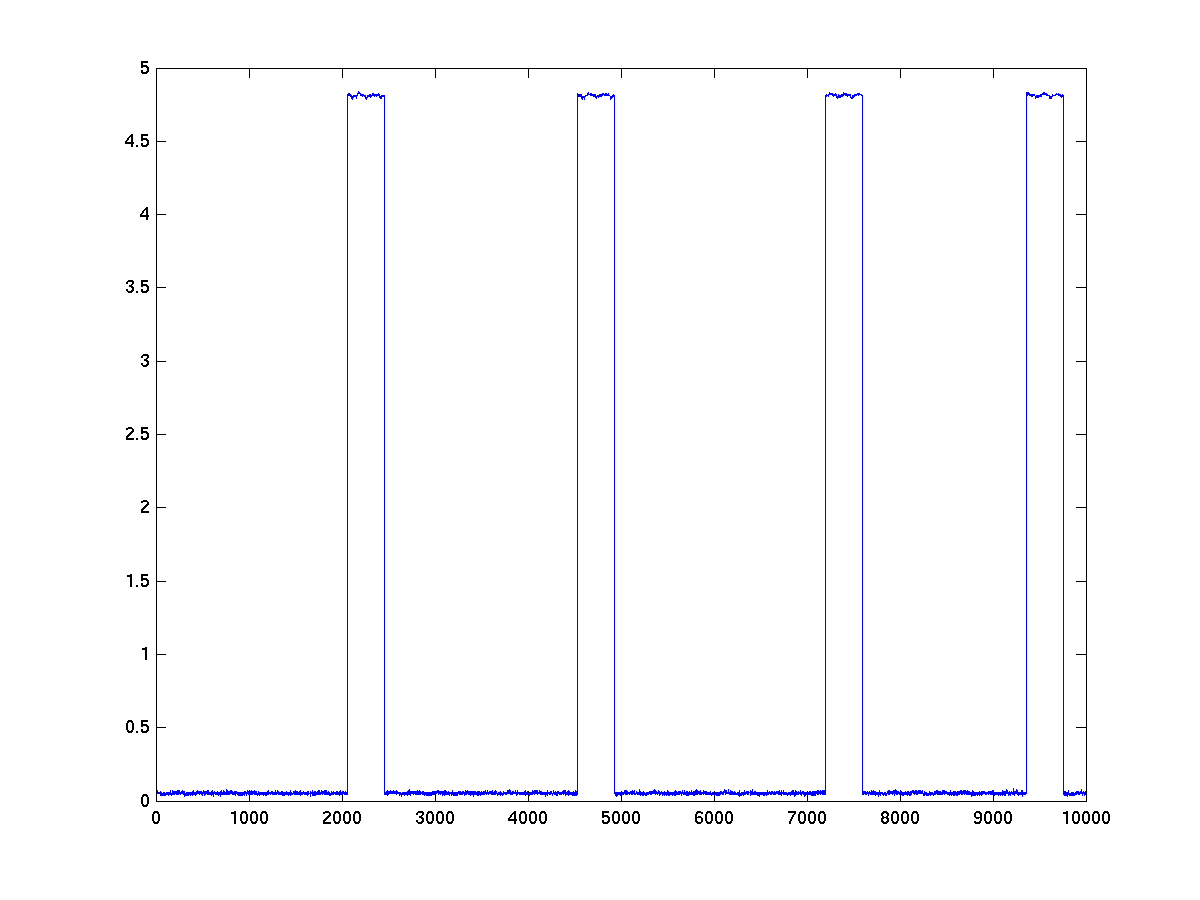zooming in even more using the MATLAB figure magnifying glass on the top left corner of one trigger event

``````figure;
plot(data(161,1:10000,'.-');
``````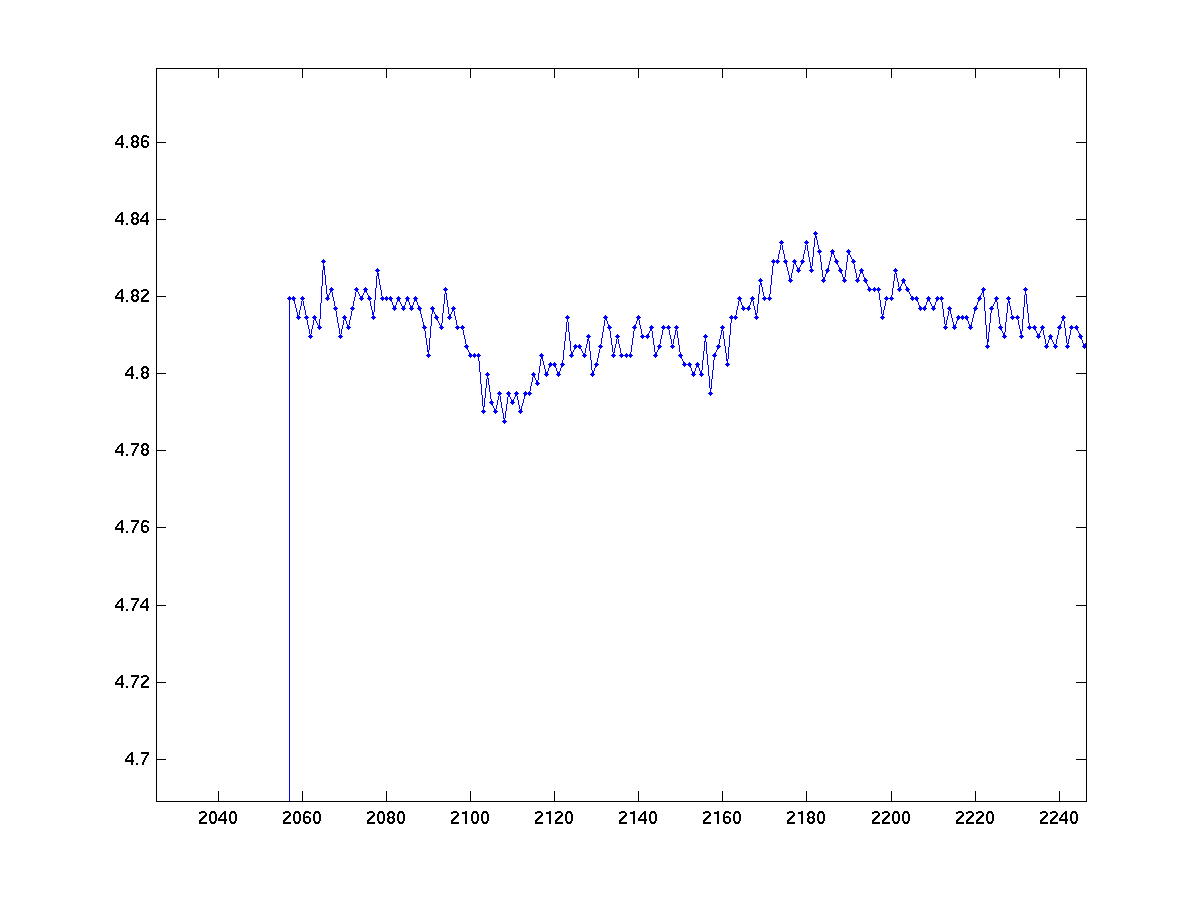We can no clearly see that a single trigger is composed of several samples of a ‘high’ signal, and that its ‘noisy’ due to the fact that it is a digitized analogue signal. Its important to make sure one has a minimal and consistent duration of the trigger signal so it can be sampled reliable for the detection of events.

According to the example here we can now determine

1. trigger 161 is indeed a trigger channel
2. the up-state around 2.5^10 units is the trigger-on state
3. halfway between 0 and 2.5^10 would therefore be a good threshold for flank detection.

Together with our knowledge of the experimental design and stimulus equipment we also know that the stimulus is presented at the moment of up-flank of the trigger channel. Lets say the same turned out to be the case in trigger channels 162 to 166, we can make a trialfunction to read in the events.

For example

``````function [trl, event] = mytrialfun(cfg)

% read the header information (including the sampling rate) and the events from the data
event = ft_read_event(cfg.dataset, 'chanindx', 161:166, 'threshold', 1e4, 'detectflank', 'up');

% search for "trigger" events according to 'trigchannel' defined outside the function
value  = [event(find(strcmp(cfg.trialdef.trigchannel, {event.type}))).value]';
sample = [event(find(strcmp(cfg.trialdef.trigchannel, {event.type}))).sample]';

% creating your own trialdefinition based upon the events
trl = [];
for j = 1:length(value);
trlbegin = sample(j) - round(cfg.trialdef.prestim  * hdr.Fs);
trlend   = sample(j) + round(cfg.trialdef.poststim * hdr.Fs);
offset   = -round(cfg.trialdef.prestim  * hdr.Fs);
newtrl   = [ trlbegin trlend offset];
trl      = [ trl ; newtrl];
end
``````

We can then proceed in the standard way of defining trials and reading data as follows. Note that except for the MEG channels which are prefixed by ‘AG’, the labels of the channels are just a string representation of the index number of the channel (starting with 1). The labels of our trigger channels are therefor ‘161’, ‘162’ and ‘163’, etc. Also realize that how the Yokogawa system is recording events through individual (analogue) channels, one is in most cases limited to one event ‘type’ per channel. In FieldTrip the channel label (which is a string of the index number) is given in the .type field of every event. In this example this is used by feeding `cfg.trialdef.trigchannel = '161'`{matlab} into the trialfunction

``````cfg = [];
cfg.dataset                 = data.sqd;
cfg.hpfilter                = 'yes';
cfg.hpfreq                  = 1;
cfg.continuous              = 'yes';
cfg.trialdef.prestim        = 1; % in seconds, time before the trigger
cfg.trialdef.poststim       = 1; % in seconds, time after the trigger
cfg.trialdef.trigchannel    = '161';
cfg.trialfun                = 'mytrialfun';

% add the "trl" array to the cfg
cfg           = ft_definetrial(cfg);

preproc                     = ft_preprocessing(cfg);
``````

As an alternative to the above procedure, it might be possible in some situations to use the generic ‘ft_trialfun_general’ to define the epochs-of-interest. This is the case if numeric triggers are sent from the presentation equipment to the MEG acquisition machine, and if the binary representation of these numeric triggers are represented as synchronous pulses on several TTL trigger channels. For instance, a trigger with a the value ‘5’ is then represented as a synchronous pulse on te first and the third trigger channel. The crux is then to instruct the ft_redefinetrial function to combine the binary representation of the triggers back into a compound trigger, using using the option `cfg.trialdef.combinebinary=true`{matlab}. Also, you may want to consider to specify a ‘trigshift’ in order to make the trigger detection a bit more robust for small asynchronies between the individual binary trigger pulses. An example cfg for this scenario could look a bit like the following:

``````cfg = [];
cfg.dataset  = '03AS_01.con';
cfg.trialdef.eventvalue = 4;
cfg.trialdef.prestim    = 1;
cfg.trialdef.poststim   = 1;
cfg.trialfun = 'ft_trialfun_general';
cfg.trialdef.chanindx = 225:232;
cfg.trialdef.threshold = 2.5; % this is a meaningful value if the pulses have an amplitude of ~5 V
cfg.trialdef.eventtype = 'combined_binary_trigger'; % this will be the type of the event if combinebinary = true
cfg.trialdef.combinebinary = 1;
cfg.trialdef.trigshift = 2; % return the value of the combined pulse 2 samples after the on-ramp (in case of small staircases)
cfg = ft_definetrial(cfg);
``````

## Creating a custom channel layout

Many FieldTrip plotting functions, such as `ft_topoplotER`, make use of a channel layout that specifies the position of the MEG channels. For some systems, FieldTrip includes template layout files and these are loaded when data are plotted. For Yokogawa systems, however, there exist many different layouts across labs, making it impractical to have a layout file available for every system in FieldTrip. Moreover, for some systems with the same number of channels (e.g., Yokogawa systems with 160 channels), the layouts are slightly different across systems/labs.

Rather than using a template layout, it is also possible to create a layout specifically for your system using your own data. In the example below, `ft_read_sens` is used to read in the position of the sensors from .con or .sqd data files. The ft_prepare_layout function is then used to define a layout based on these positions.

In this example, the CTF151 helmet “outline” and “mask” are added to the KIT/Yokogawa layout to replace the standard sphere-with-triangle-for nose. For visualization, the position of the sensors are stretched and scaled to match the helmet and extend a bit beyond the ears. You can change these parameters if your own layout do not look correct yet. The last two channels in the layout (`COMNT` and `SCALE`) are excluded from the scaling and shifting, so that they stay in the same position.

``````% read the position of the sensors from the data

% prepare the custom channel layout
cfg                         = [];
layout                      = ft_prepare_layout(cfg);
sel                         = 1:(length(layout.label)-2); % the last two are COMNT and SCALE

% scale & stretch the position of the sensors
layout.pos(sel,:)           = layout.pos(sel,:) * 1.05;
layout.pos(sel,2)           = layout.pos(sel,2) * 1.08 + 0.02;

cfg                         = [];
cfg.layout                  = 'CTF151_helmet';
ctf151                      = ft_prepare_layout(cfg);

layout.outline              = ctf151.outline;

% plot the custom layout
figure;
ft_plot_layout(layout, 'box', 1);
``````

The `layout` structure may then be passed in `cfg.layout` to plotting functions such as ft_topoplotER and ft_multiplotER.

## Coordinate system coregistration

Each of the scanners used in neuroimaging research in principle has its own hardware-based coordinate system: the MRI has a coordinate system that relates to the bore, the MEG has a coordinate system that relates to the dewar, and the polhemus tracker has a coordinate system relative to the transmitter (the two-inch gray cube). Using a combination of the three systems (MRI, MEG, Polhemus) we try to relate the neuronal activity in the MEG to an anatomical location in the MRI.

The general principle of coordinate system coregistration is to measure the same fiducial locations as points of reference with the different systems. Often, but not always, the fiducial locations are chosen to match clearly defined anatomical landmarks, such as the nasion (the bridge of the nose) and points that relate to the ear (e.g., pre-auricular points, mastoids, the ear canal). To ensure that the same points can be recorded with the different types of scanners, the points are marked

1. MRI using vitamine E capsules or another oily substance that has a large MR contrast
2. MEG using coils through which an alternating electrical current can be passed, resulting in a small but well-localized magnetic field
3. Polhemus using a felt-tip pen

In general MEG analyses are performed in a “head coordinate system” that relates to the anatomical landmarks. To get a clear picture of the head coordinate system, you should consider how the location of the origin (i.e. the point [0 0 0]) and the direction of the axes of the coordinate system (i.e. the x-, y- and z-axis) are defined in relation to the anatomical landmarks. For example, the origin of the coordinate system can be defined exactly between the two ears and the x-axis (or the y-axis) can be defined to point towards the nasion. Note that different hardware manufacturers and software packages use different conventions, e.g., the x-axis can point either to the nose (CTF) or to the right ear (Neuromag), and that different labs also use slightly different conventions for the “ear” landmarks.

Alternative to anatomically defined landmarks, it is also possible to use fiducials on other locations, as long as the position of the same fiducials can be detected with the different scanners. For the Yokogawa system three coils (MEG) or three vitamine capsules (MRI) can used that are placed on the forehead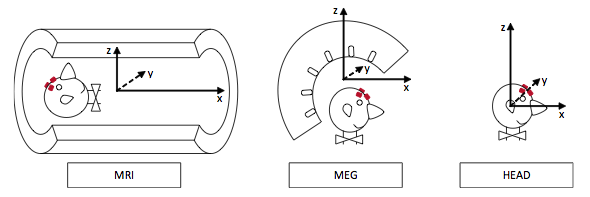In the frequently asked questions you can find an overview of the conventions for the coordinate systems that are used by various MEG and MRI systems/software.

### MEG Dewar Coordinate System

Unlike other systems, the Yokogawa system software does not automatically analyze its sensorlocations relative to fiducial coils. Instead the positions of the fiducial points are saved in an external textfile - in the helmet’s own coordinate system - using the property menu of the YOKOGAWA MEG-VISION software. Origin of the coordinate system is at the center of the helmet, where Z+ is towards the top of the head, X+ is towards the nose, Y+ is towards the left.If you used standard fiducial locations (nasion, left/right ear) for the MEG coils as well as during MR scanning (using e.g., vitamin pill) the procedure of co-registration is relatively standard after this. However, in the following example dataset, co-registration points on the forehead were used.

### Aligning the MRI with the head coordinate system

``````ft_hastoolbox('spm8',1);
``````

First we use ft_volumerealign to locate the nasion and the auricular points (using the n/l/r keyboard input). This will return the anatomical MRI in head coordinates.

``````cfg             = [];
cfg.method      = 'interactive';
cfg.coordsys    = 'ctf';
mri_aligned     = ft_volumerealign(cfg,mri);
``````

### Aligning the MEG with the head coordinate system

With the MEG we have determined the position of the forehead marker coils relative to the MEG dewar. Using ft_sourceplot we can determine the coordinates of the forehead markers in the anatomical MRI.

``````cfg             = [];
cfg.method      = 'ortho';
cfg.interactive = 'yes';
ft_sourceplot(cfg,mri_aligned);
``````

By clicking in one of the panels of the figure, the position of the crosshair will be updated and the three orthogonal slices will be redrawn. If you look in the MATLAB command-line window, you will also see that the current position of the crosshair is displayed. You should see something like this every time you click

``````click with mouse button to reposition the cursor
press n/l/r on keyboard to record a fiducial position
press q on keyboard to quit interactive mode
voxel 2152181, indices [91 173 55], ctf coordinates [0.0 47.0 -18.0] mm
``````

The location of the crosshair is expressed in voxel indices and in mm units relative to the head coordinate system that you determined previously with ft_volumerealign

You now have to find the three forehead markers in the MRI and write down the coordinates of these three fiducials in the head coordinate system.Using the three fiducial points expressed both in head coordinates and in MEG dewar coordinates, we can transform all other spatial locations (i.e. the sensors) from dewar coordinates to head coordinates. We have determined the positions relative to the head coordinate system using the MRI, so we now need the fiducial positions that were recorded relative to the MEG dewar coordinate system.

The MEG fiducial positions are stored in an ASCII text file that you can open in the MATLAB editor.

``````edit  marker-coregis.txt
``````

Using the MRI fiducial positions expressed in head coordinates, and the MEG fiducial positions expressed in dewar coordinates, we can transform the MEG sensor positions from dewar into head coordinates.

``````% read the gradiometer definition from file, this is in dewar coordinates
``````

Alternative to reading the gradiometer definition from the raw data file, you can also obtain the gradiometer definition after ft_preprocessing, ft_timelockanalysis or ft_freqanalysis: the data structures resulting from those functions contain the “grad” field which corresponds to the gradiometer definition from the original raw file.

``````% add the fiducials (expressed in dewar coordinates) to the gradiometer definition

% the configuration for FT_SENSORREALIGN should specify the three fiducials in
% head coordinates as obtained from the aligned MRI using FT_SOURCEPLOT
cfg = [];
cfg.method = 'fiducial';
cfg.template.pnt = [
];
cfg.template.label = {
'fid1_label'
'fid2_label'
'fid3_label'
};
``````

## Example: Sourceanalysis

The realigned anatomical MRI can be segmented to aid in the construction of the volume conduction model can be made.

``````cfg           = [];
mri_segmented = ft_volumesegment(cfg, mri_aligned);
% it is convenient to keep the original anatomical MRI with the segmentation
mri_segmented.anatomy = mri_aligned.anatomy;
``````

We can now make the headmodel

``````cfg             = [];
cfg.sourceunits = 'mm'; %this option will be depricated
cfg.mriunits    = 'mm'; %this option will be depricated
vol             = ft_prepare_singleshell(cfg, seg);
``````

Because the gradiometer coordinates are in cm, and the MRI derived geometrical objects in mm, we have to put those in cm also.

``````vol_cm          = ft_convert_units(vol,'cm');
coil_cm         = ft_convert_units(coil_sens, 'cm');
``````

Plot sensors, fiducials and headmodel to doublecheck

``````figure;
plot3(coil_cm.pnt(:,1), ...
coil_cm.pnt(:,2), ....
coil_cm.pnt(:,3),'r.','MarkerSize',25);
hold on
xlim([-15,15]);
zlim([-15,15]);
ylim([-15,15]);
xlabel('X');
ylabel('Y');
zlabel('Z');
camlight left
camlight left
``````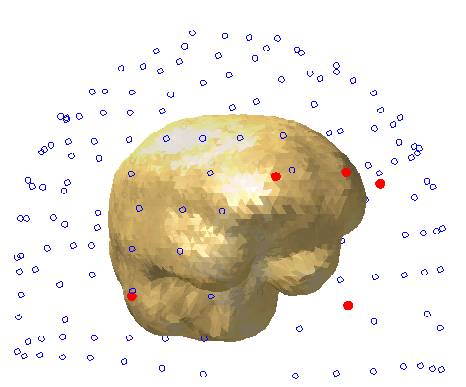### Freqanalysis

Now we’re at it, lets make a start using the above frequency data / geometrical objects for beamforming sourceanalysis.

Redefine trials to make baseline estimate

``````cfg             = [];
cfg.toilim      = [-.8 -.3];
data_BL         = ft_redefinetrial(cfg,ft_data);

cfg             = [];
cfg.method      = 'mtmfft';
cfg.output      = 'powandcsd';
cfg.foi         = 1:20;
cfg.taper       = 'dpss';
cfg.tapsmofrq   = 2;
freq_BL         = ft_freqanalysis(cfg,data_BL);
``````

Do freqanalysis on left/right cue conditions

``````cfg             = [];
cfg.toilim      = [.5 1];
cfg.trials      = find(ft_data.trialinfo(:,1) == 3 | ft_data.trialinfo(:,1) == 4);
data_stiml      = ft_redefinetrial(cfg,ft_data);

cfg             = [];
cfg.method      = 'mtmfft';
cfg.output      = 'powandcsd';
cfg.foi         = 1:20;
cfg.taper       = 'dpss';
cfg.tapsmofrq   = 2;
freq_stiml      = ft_freqanalysis(cfg,data_stiml);

% same for right-cue
cfg             = [];
cfg.toilim      = [.5 1];
cfg.trials      = find(ft_data.trialinfo(:,1) == 1 | ft_data.trialinfo(:,1) == 2);
data_stimr      = ft_redefinetrial(cfg,ft_data);

cfg             = [];
cfg.method      = 'mtmfft';
cfg.output      = 'powandcsd';
cfg.foi         = 1:20;
cfg.taper       = 'dpss';
cfg.tapsmofrq   = 2;
freq_stimr      = ft_freqanalysis(cfg,data_stimr);
``````

Its always good to use as much data as possible for your common filter

``````data_common     = ft_appenddata([], data_BL, data_stimr, data_stiml);

cfg             = [];
cfg.method      = 'mtmfft';
cfg.output      = 'powandcsd';
cfg.foi         = 1:20;
cfg.taper       = 'dpss';
cfg.tapsmofrq   = 2;
freq_common     = ft_freqanalysis(cfg,data_common);
``````

Lets check out the sensor level first

``````freq_diff = freq_stimr;
freq_diff.powspctrm = (freq_stimr.powspctrm - freq_stiml.powspctrm) ./ (freq_stimr.powspctrm + freq_stiml.powspctrm) ;

freq_r_bl = freq_stimr;
freq_r_bl.powspctrm = (freq_stimr.powspctrm ./ freq_common.powspctrm)  ;

freq_l_bl = freq_stiml;
freq_l_bl.powspctrm = (freq_stiml.powspctrm ./ freq_common.powspctrm)  ;

cfg             = [];
lay             = ft_prepare_layout(cfg, data_common);

cfg             = [];
cfg.layout      = lay;
cfg.xlim        = [10 10];
cfg.zparam      = 'powspctrm';

figure; ft_topoplotTFR(cfg, freq_r_bl);
% figure; ft_topoplotTFR(cfg, freq_diff);
% figure; ft_topoplotTFR(cfg, freq_l_bl);
``````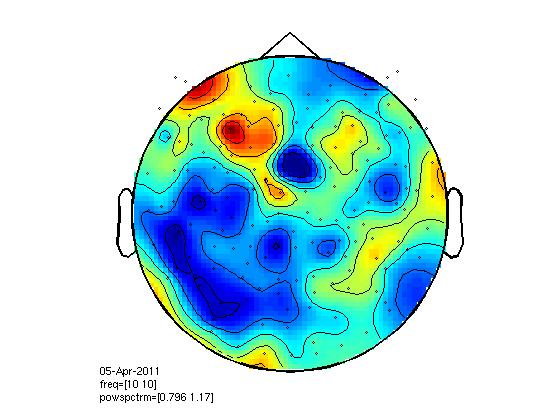### Calculate spatial filter

compute common spatial filter

``````cfg = [];
cfg.xgrid      = -15:0.5:15;
cfg.ygrid      = -15:0.5:15;
cfg.zgrid      = -15:0.5:15;
cfg.inwardshift     = -2;
cfg.channel         = {'all'}; % also MEGREF channels
cfg.reducerank      = 2;
cfg.frequency       = 10;
cfg.method          = 'dics';
cfg.projectnoise    = 'yes';
cfg.keepfilter      = 'yes';
cfg.feedback        = 'no';
cfg.realfilter      = 'yes';
cfg.lambda          = '0.005%';
source_common       = ft_sourceanalysis(cfg, freq_common);
``````

Project cue condition through common spatial filter

``````cfg = [];
cfg.sourcemodel.pos        = source_common.pos;
cfg.sourcemodel.filter     = source_common.avg.filter;
cfg.channel         = {'all'};
cfg.reducerank      = 2;
cfg.frequency       = 10;
cfg.method          = 'dics';
cfg.projectnoise    = 'yes';
cfg.keepfilter      = 'no';
cfg.feedback        = 'no';
cfg.realfilter      = 'yes';
cfg.lambda          = '0.005%';

source_left         = ft_sourceanalysis(cfg, freq_stiml);
source_left.unit    = 'cm';
source_left.dim     = source_common.dim;

source_right        = ft_sourceanalysis(cfg, freq_stimr);
source_right.unit   = 'cm';
source_right.dim    = source_common.dim; %zou ook niet hoeve he?!?

source_diff         = source_right;
source_diff.avg.pow = (source_left.avg.pow - source_right.avg.pow) ./  (source_left.avg.pow + source_right.avg.pow) ;

source_left_bl          = source_left;
source_left_bl.avg.pow  = source_left_bl.avg.pow ./ source_common.avg.pow;

source_right_bl         = source_right;
source_right_bl.avg.pow = source_right_bl.avg.pow ./ source_common.avg.pow;
``````

### Plotting results

Interpolate for plotting on MRI

``````cfg                 = [];
cfg.method          = 'linear';
% source_left_int     = ft_sourceinterpolate(cfg,source_left,mri_aligned);
% source_right_int    = ft_sourceinterpolate(cfg,source_right,mri_aligned);
% source_diff_int     = ft_sourceinterpolate(cfg,source_diff,mri_aligned);
% source_left_bl_int  = ft_sourceinterpolate(cfg,source_left_bl,mri_aligned);
source_right_bl_int = ft_sourceinterpolate(cfg,source_right_bl,mri_aligned);
``````

Plot results

``````figure;
cfg                 = [];
cfg.method          = 'ortho';
cfg.interactive     = 'yes';
cfg.projmethod      = 'nearest';
cfg.anaparameter    = 'anatomy';
cfg.funparameter    = 'avg.pow';
cfg.funcolorlim     = [0 2];
cfg.colorbar        = 'yes';
ft_sourceplot(cfg,source_right_bl_int);
``````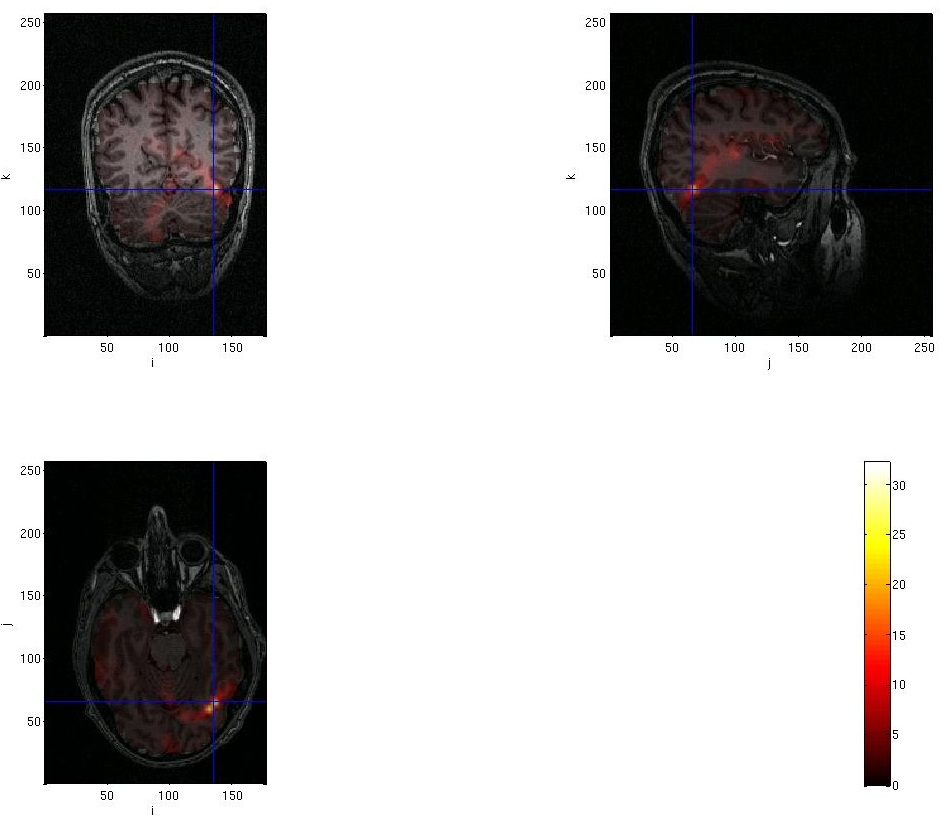Thanks to Akiko Ikkai for contributing her data for making this page.